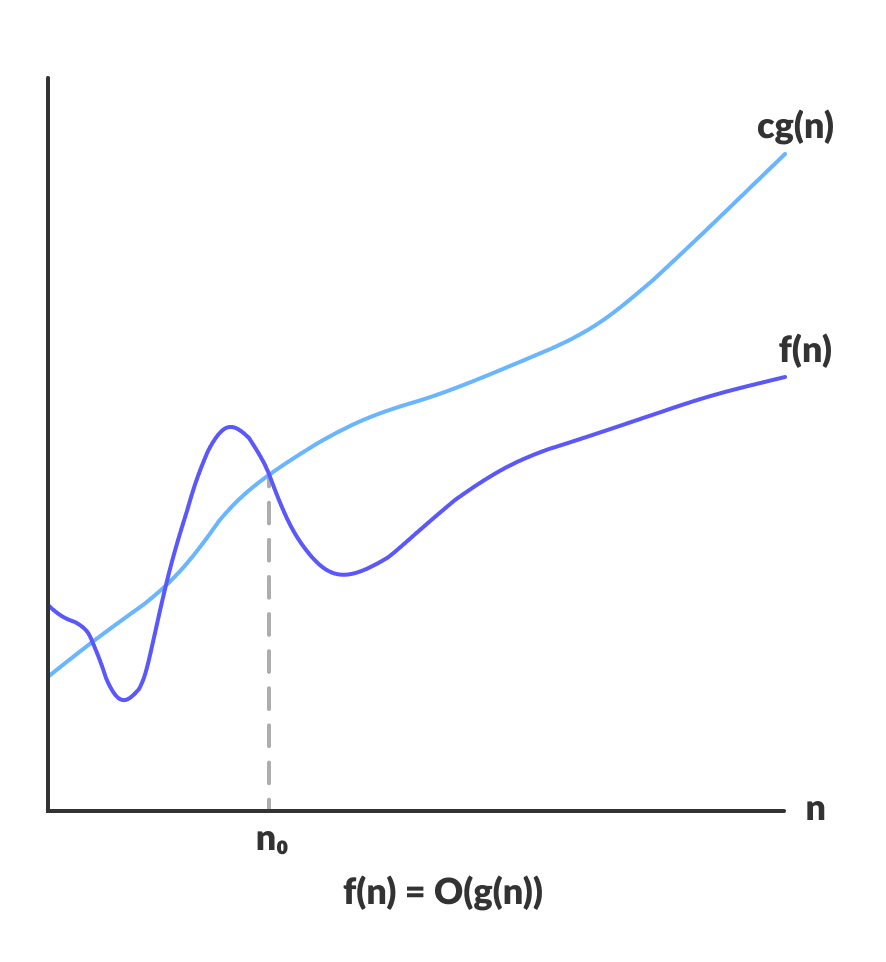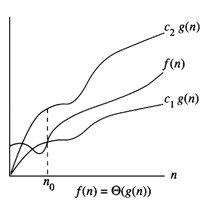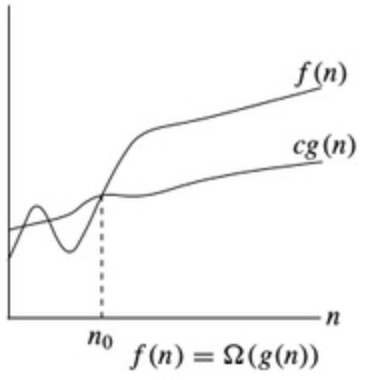# Time Complexity

## Introduction

Time complexity plays a vital role while writing the solutions for a particular problem. What do you mean by time complexity?.

Depending on the number of elements, the time the code or the algorithm takes to run is the time complexity.

As the definition implies, the time required is dictated by the number of iterations of one-line statements within the code. We can say that every piece of code we write takes time to execute—the lesser the time complexity, the faster the execution.

The time for a program to run does not depend entirely on the efficiency of code, and it’s also dependent on the processing power of a PC.

To compare the space and time complexity of the two algorithms, we will use Asymptotic Analysis. It figures out that how the performance changes as the input size increases.

Big-oh Notation(O) - Worst case

Big-oh is a formal method of expressing the upper bound of an algorithm’s running time.

It measures the longest time it could take for the algorithm to complete.

More formally, for non-negative functions, f (n) and g(n), if there exists an integer n0 and a constant c > 0 such that for all integers n > n0, f(n) < c * g(n).

Then, f (n) is the big-oh of g(n). This is denoted as f (n) O(g(n)), i.e., the set of functions which, as n gets large, grow faster than a constant time f (n).Theta-Notation(Θ) - Average case

This notation bounds a function within a constant factor.

We say f(n) = g(n) if there exist positive constants n0, c1, and c2 in such a way that 0 ≤ c1g(n) ≤ f(n) ≤ c2g(n) for all n ≥ n0.Pic from cs.fsu.edu.

Big Omega (Ω) - Best case

This notation gives a lower bound or the best case for a function within a constant factor.

We will write f(n) = g(n))when there are positive constants n0 and c such that 0 ≤ cg(n) ≤ f(n) for all n ≥ n0.Pic from the medium.com

It will be more transparent when we see various examples of different time complexities.

## Example 1:

``````public static void main(String[] args) {

System.out.println("Coding Ninjas");

}``````

Output

``Coding Ninjas``

As you can see, the above example is not based on the input size n, which is why the time complexity taken by this code will be O(1).

O(1) means a constant amount of time is required to execute the code.

## Example 2:

``````public static void main(String[] args) {

int n=3;

for(int i=0;i<n;i++)
System.out.println("Coding Ninjas");

}``````

Output

``````Coding Ninjas
Coding Ninjas
Coding Ninjas``````

In the above example, we have taken input n=3, applying the loop till input size n. ‘Coding Ninjas’ will be printed according to the input size given.

Therefore, this has a time complexity of O(n).

## Example 3:

``````public static void main(String[] args) {

int n=3;

for(int i=0; i < n; i++) {

for(int j=0; j < n;j++) {

System.out.println("Coding Ninjas");
}
}
}``````

Output

``````Coding Ninjas
Coding Ninjas
Coding Ninjas
Coding Ninjas
Coding Ninjas
Coding Ninjas
Coding Ninjas
Coding Ninjas
Coding Ninjas``````

In the above example, we have taken input n=3, applying the nested loops to input size n. ‘Coding Ninjas’ will be printed n*n times.

Therefore, this has a time complexity of O(n^2).

## Example 4:

``````public static void main(String[] args) {

int n=6;
int arr[]=new int[] {1,2,3,4,5,6};
int low=0,high=n-1;
int target=3;

while(low <= high) {

int mid = (low + high) / 2;
if (target < arr[mid])
high = mid - 1;
else if (target > arr[mid])
low = mid + 1;
else
break;
}
}``````

This is the code for binary search, which follows the divide and conquer approach. The algorithm’s running time is proportional to the number of times N can be divided by 2.

Therefore, the time complexity of this algorithm will have a Logarithmic, i.e., O(log n).

## Example 5:

``````void quicksort(int list[], int left, int right)
{
int pivot = partition(list, left, right);
quicksort(list, left, pivot - 1);
quicksort(list, pivot + 1, right);
}``````

The above example is the logic of the QuickSort algorithm which also follows the divide and conquer approach, just like Binary search, but here, we repeat the iteration N times.

Hence time complexity will be n*log( n ).

## FAQ’S

1). What is the difference between Space and Time Complexity?
Time complexity is the amount of time the code is required to be executed. Whereas space complexity is the amount of space needed by the code.

2). Rank the following by growth rate: n, 2 log n, log n, log (log n), log2 n, (log n) log n, 4, (3/2)^n, n! ?

Rank in increasing order of growth rate is 4,  log (log n), log n, log2 n, (log n)log n, 2 log n, n, n!, (3/2)^n.

## Key Takeaways

This blog has discussed how time complexity deals with the time required to execute a program.

We learned to compute time complexity and how important it is to keep in mind to write good code.

Also, don’t forget to practice the wide variety of DSA problems frequently asked in interview rounds readily available on CodeStudio

Happy coding!